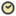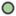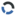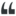Dev:Types

 Type Description 01Bool a boolean value; true or false. Represented in Studio as a checkbox. 02Integer an integer value; a whole number. -3 or 0 or 2 are examples. 03Real a real number. -3.0, 0.0, 2.0 and 3.1415 are examples. 04Angle a real number that is interpreted as an angle. 05Time a time, displayed in days, hours, minutes, seconds and frames. 06Vector a 2d vector. usually represented as two Reals representing X and Y values. 07Color a color. Usually represented as Red/Green/Blue/Alpha values. 08Segment a single bezier curve segment. Defined by 4 Vectors, representing 2 Vertices and 2 Tangents. 09SplinePoint a point in a Spline. Defined by 6 parameters (a Vector defining its location, a Real defining its width, another Real defining its origin, a Bool defining whether its tangents are split or not, and two Vectors defining the direction of its tangents (only the first of which is used if the tangents aren't split). 10List A list. For example, an outline's Vertices parameter is simply a list of SplinePoint valuenodes. 11Canvas A collection of layers. When you group layers, you're creating a new Group Layer called, by default "Group". The group layer has a parameter called 'Canvas' with type 'Canvas' and its value is the group of layers you've grouped. 12String a string of characters, for example "Hello Mum!" 13Gradient a range of colors (a mapping from a real parameter to a color). Can be defined in many ways, but is usually editing using the gradient editor.